# NOT Gate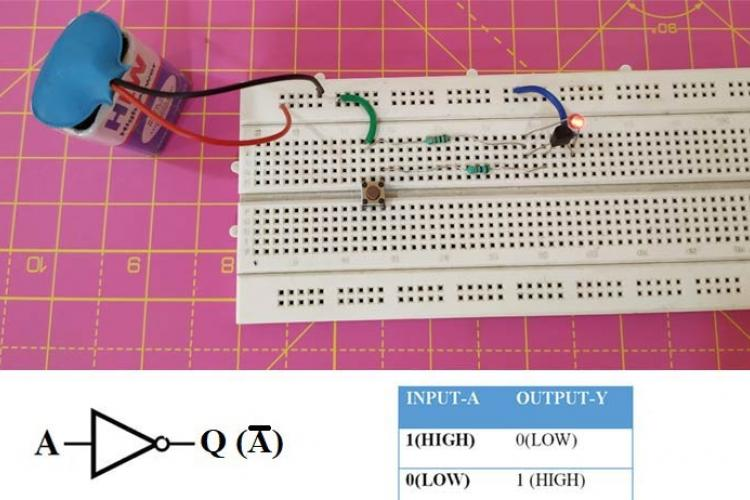Designing NOT Gate using TransistorsAn Integrated Circuit or IC is a combination of many small circuits in a small package that together performs a common task.…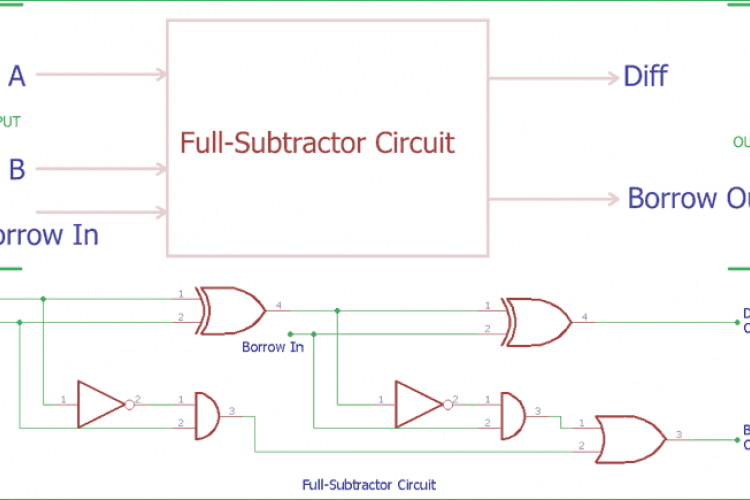Full Subtractor Circuit and Its ConstructionIn the previous tutorial of Half Subtractor Circuit, we had seen how computer use single bit binary numbers 0 and 1 for…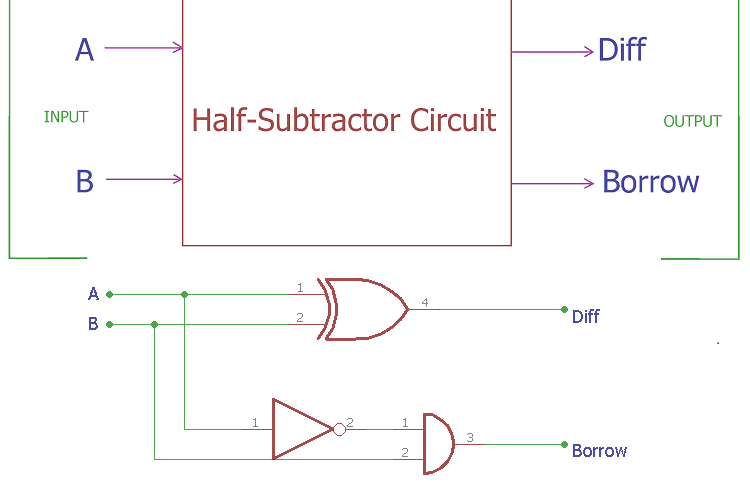Half Subtractor Circuit and Its ConstructionIn previous tutorials, we have seen how computer use binary numbers 0 and 1 and by using an adder circuit computer will add…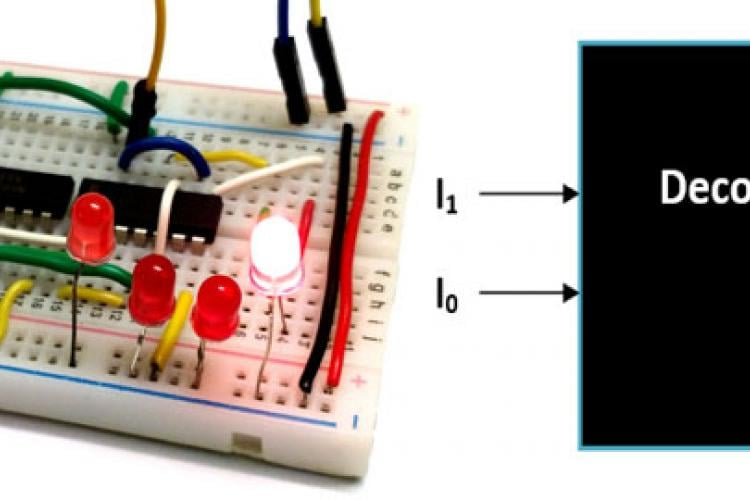Binary DecodersDecoder is type of combinational Circuit which decodes a small bit value into large bit value. It is normally used in…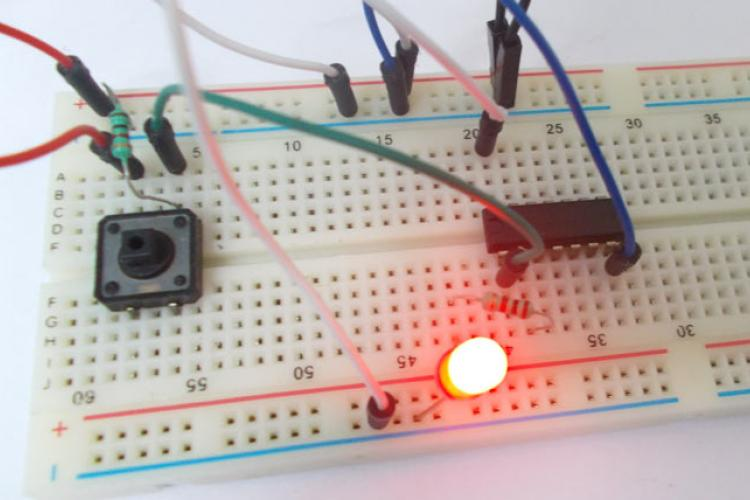NOT GateNOT gate is a digital logic gate, designed for arithmetic and logical operations, every electronic student must have studied…